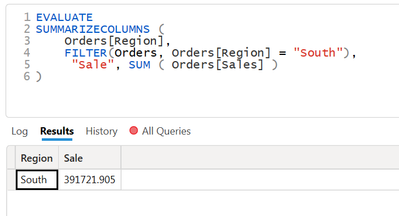cancel
Showing results for
Did you mean:## How to create calculated table with numeric condition.

I want to create a calculated table with Region and Sales where the sale is greater than 500,000.

This is the DAX I created to generate the table.I can also create a text based filter condition, but I am unable to create the numerical condition.DAX

SUMMARIZECOLUMNS (
Orders[Region],
FILTER(Orders, Orders[Region] = "South"),
"Sale", SUM ( Orders[Sales] )
)

1 ACCEPTED SOLUTIONSuper User

Hi,

I am not sure if I understood your question correctly, but please try below whether it suits your requirement.

``````New table =
FILTER (
SUMMARIZECOLUMNS ( Orders[Region], "@Sale", SUM ( Orders[Sales] ) ),
[@Sale] >= 500000
)
``````

If this post helps, then please consider accepting it as the solution to help other members find it faster, and give a big thumbs up.

2 REPLIES 2Super User

Hi,

I am not sure if I understood your question correctly, but please try below whether it suits your requirement.

``````New table =
FILTER (
SUMMARIZECOLUMNS ( Orders[Region], "@Sale", SUM ( Orders[Sales] ) ),
[@Sale] >= 500000
)
``````

If this post helps, then please consider accepting it as the solution to help other members find it faster, and give a big thumbs up.Thank you @Jihwan_Kim for giving the solution it worked.

Meanwhile I also found this alternative that works too.

``````SUMMARIZECOLUMNS (
Orders[Region],
FILTER ( VALUES ( Orders[Region] ), [Total Sale] > 500000 ),
"Total Sale", Sum(Orders[Sales])
)``````